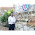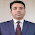## Sunday, October 18, 2009

### Excel Dates : Leap Year or Not a Leap Year

Excel follows Gregorian calendar which was first established in 1582 by Pope Gregory XIII.

### To determine whether a year is a leap year, follow these steps:

`  1. If the year is evenly divisible by 4, go to step 2. Otherwise, go to step 5.   2. If the year is evenly divisible by 100, go to step 3. Otherwise, go to step 4.   3. If the year is evenly divisible by 400, go to step 4. Otherwise, go to step 5.   4. The year is a leap year (it has 366 days).   5. The year is not a leap year (it has 365 days). `

Following formula gives the results based on above steps. This considers that you have stored year in Cell A1 of your worksheet

=IF(OR(MOD(A1,400)=0,AND(MOD(A1,4)=0,MOD(A1,100)<>0)),"Leap Year", "NOT a Leap Year")

Since excel has all these calculations built in , you can just test the last day of the February month and decide based on day value. If it is 29 then Leap Year else NOT a Leap Year.

Following formula gives the results based on above steps. This considers that you have stored year in Cell A1 of your worksheet

=IF(DAY(DATE(A1,3,0))=29,"Leap Year","NOT a Leap Year")

First Formula returns 1900 as "NOT a Leap Year" but second Formula will return it as "Leap Year". This is due to bug in excel which considers 1900 as a Leap Year though it was not a leap year.

This is why I will recommend to use second Formula in excel to determine a Leap Year since it takes care of any bug that exists in excel

### VBA Function for Leap Year Test:

`Public Function IsLeapYear(ByVal YY As Long) As Boolean    IsLeapYear = Day(DateSerial(YY, 3, 0)) = 29End Function`

## Leap Year , leap year test , leap year check , check leap year , Leap Year in Excel

1.Some people may not see the date specification part of this formula...

=IF(DAY(DATE(A1,3,0))=29,"Leap Year","NOT a Leap Year")

as referring to a February date. An alternative test which makes this a little clearer would be this...

=IF(MONTH(DATE(A1,2,29))=2,"Leap Year","NOT a Leap Year")

Another way we could do this test is as follows...

=IF(ISNUMBER(--(A1&"-02-29")),"Leap Year","NOT a Leap Year")

The only reason I mention this version is that the test uses only one function call as opposed to the two used in the previous versions. Theoretically, this should make it more efficient, although there is a loss in efficiency due to the concatenation. The VBA equivalent code for these two approaches would be...

IsLeapYear = Month(DateSerial(YY, 2, 29)) = 2

and...

IsLeapYear = IsDate("2/29/" & YearIn)

Rick Rothstein (MVP - Excel)

2.Hello Rick

3.Kindly advise how we convert the date 13.08.2012 to 08/13/2012 format.

1.Right Click
Click Format Cells
Click Custom
Type dd/mm/yyyy

4.yogesh ji,

i need help to create a order form in excel,by merging to excel file,
for both example file i want to mail you,
if possiable kindly provide your mail id, or other way to post that files,

regards

harish nebhwani

sultanate of oman

5.=IF(MOD(A1,4)=0,"Leap Year","Non Leap Year")

6.yogesh sir, pl help I want to numeric date of birth in text in exel. for example 12/10/2016 conver into twelve october two thousand sixteen. ceate formulae in exel

7.Your post are inspiring. I hope you will write more such post. Each of your post brings a lot of good.
Digital Marketing Course in Kolkata